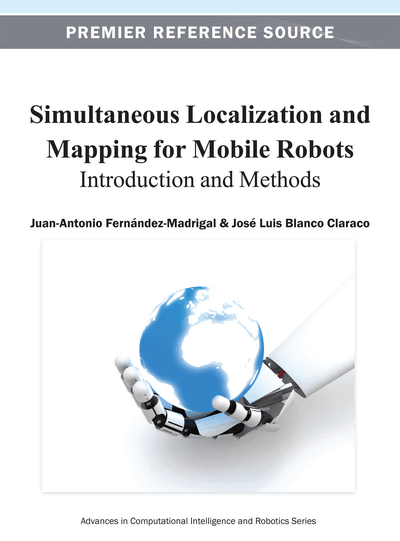# Statistical Bases

Copyright: © 2013 |Pages: 29
DOI: 10.4018/978-1-4666-2104-6.ch004
OnDemand PDF Download:
Available
\$37.50
No Current Special Offers

## Abstract

This is the fourth and last chapter of the first section. As chapter 3 introduced the mathematical tools of probability theory needed to understand all the concepts in the book, chapter 4 does the same concerning statistics. It fills the gap between probability theory and real data coming from stochastic processes, highlighting the great amount of potential applications of the different fields of statistics—particularly estimation theory—in state-of-the-art science and engineering. Topics covered in this chapter include the fundamental tools needed in probabilistic robotics: probabilistic convergence, theory of estimators, hypothesis tests, etc. Special stress is on recursive Bayesian estimators, due to their central role in the problems of probabilistic robot localization and mapping.
Chapter Preview
Top

## Chapter Guideline

• You will learn:

• o

In which ways the uncertainty in a set of observations may converge to a given probabilistic model as the number of observations increases.

• o

What are the sample mean and sample variance, and their difference with the theoretical mean and variance of a distribution.

• o

Why having many observations is better than having few.

• o

Why the normal distribution has such a prevalent role in science and in nature.

• o

What is an estimator and which of its properties shall interest us.

• o

How to construct classical and Bayesian estimators.

• o

The bases on estimating dynamic processes.

• Provided tools:

• o

The central limit theorem and the law of large numbers.

• o

The construction of maximum likelihood estimators.

• o

The method of moments for constructing estimators.

• o

The basic Bayesian estimators: MMSE, MAP, and MED.

• o

The general Bayesian framework for estimating dynamic Markov processes.

• Relation to other chapters:

• o

Bayesian estimators introduced in this chapter are applied to practical robotic problems in chapters 7, 8, and 9.

• o

A central part of chapter 10 deals with the concept of maximum likelihood estimation and its role in the recent approaches to robot map building in especially difficult scenarios.

Top

## 1. Introduction

Statistics is the branch of mathematics dealing with the collection, analysis, interpretation and presentation of numerical data (Merrian-Webster, 2011). It is, therefore, the science of making effective use of data acquired from stochastic processes in order to describe them, infer new information, or making predictions. Statistics is based on probability theory (the subject of chapter 3), but it is a different science, more practical and, sometimes, less abstract. Its historical development was intertwined with that of probability theory, as depicted in the previous chapter.

Statistics has its own diverse branches, each focused on the different aspects of the analysis of data. Descriptive statistics has to do with synthesizing the main characteristics of collected data, while statistical inference draws conclusions from these data in spite of their randomness. In mobile robot localization and mapping, the most relevant area is that of estimation: inferring the characteristics of the current uncertainty exposed by r.v.s from the observation of the values they yield. Other branches, such as prediction (estimation of the information that the r.v.s will expose in the future) have currently a marginal presence in mobile robotics, mostly in task or motion planning, which are outside the scope of this book.

## Complete Chapter List

Search this Book:
Reset